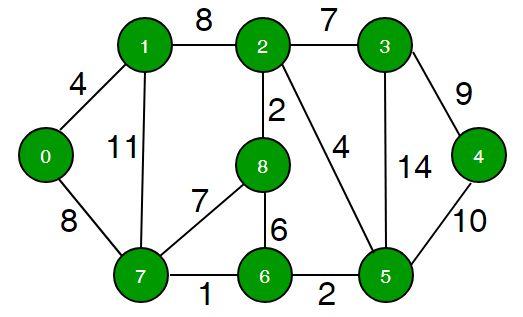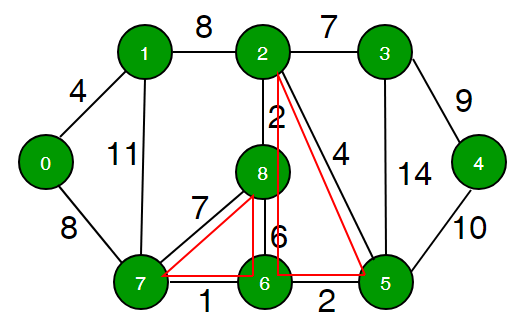# Find the minimum weight cycle in an undirected graph

Find the minimum weighted cycle in an undirected graph can be found from. An example from  is shown here to well explain this problem.An example of a weighted undirected graph fromMinimum weighed cycle : 7 + 1 + 6 = 14 or 2 + 6 + 2 + 4 = 14 

Q&A from 

Q

“Let G be a directed weighted graph with no negative cycles. Design an algorithm to find a minimum weight cycle in G that runs with a time complexity of O(|V|³).”

The above is a question I have been working on as part of my coursework. When I first read it, I immediately thought that the Floyd-Warshall algorithm would solve this problem — mainly because F-W runs in O(|V|³) time and it works for both positive and negative weighted graphs with no negative cycles. However, I soon remembered that F-W is designed to find the shortest path of a graph, not a minimum weight cycle.

Am I on the right track with this question? Would it be possible to modify the Floyd-Warshall algorithm to find a minimum weight cycle in a graph? by user1696230

A

I think you are pretty close. Once you do FW loop in O(|V|³), you have the weight of a shortest path between each pair of vertices, let’s call it D(i, j). Now the solution to our main problem is as follows: Brute force for the vertex which is gonna be in a loop, say it’s X. Also brute force on Y, which is gonna be the last vertex in the cycle, before X itself. The weight of this cycle is obviously D(X, Y) + W(Y, X). Then you just choose the one with the smallest value. It’s obviously a correct answer, because: (1) All these values of D(X,Y)+D(Y,X) represent the weight of some(maybe non-simple) cycle; (2) If the optimal cycle is some a->…->b->a, then we consider it when X=a, Y=b;

To reconstruct the answer, first you need to keep track of which X, Y gave us the optimal result. Once we have this X and Y, the only thing remaining is to find the shortest path from X to Y, which can be done in various ways. by llaki

 mentions that both Dijkstra and Floyd Warshall algorithm can be used to find the minum weighted cycle in undirected graph.

 mentions 3 algorithms used to solve this problem. Except the Digkstra and Floyd Warshall algorithms, a brute force algorithm is also mentioned here. The complexity of each algorithm is analyzed here.

 gives two ample codes used to solve the problem .

 gave a nice solution for problem 

`#include<stdio.h>#include<string.h>#include<algorithm>using namespace std;#define inf 1044266558typedef struct Point{ int x, y; Point operator - ( const Point &b ) const {  Point c;  c.x = x-b.x; c.y = y-b.y;  return c; } double operator * ( const Point &b ) const {  return x*b.y-y*b.x; }}Point;Point h, s;int n, m, ans, road;bool Jud(Point x, Point y, Point z){ if((x.x<z.x && y.x<z.x) || (x.y<z.y && y.y<z.y) || (x.x>z.x && y.x>z.x) || (x.y>z.y && y.y>z.y))  return 1; return 0;}int main(void){ int i, j, k, flag; while(scanf("%d", &n)!=EOF) {  memset(road, 62, sizeof(road));  for(i=1;i<=n;i++)   scanf("%d%d", &h[i].x, &h[i].y);  scanf("%d", &m);  for(i=1;i<=m;i++)   scanf("%d%d", &s[i].x, &s[i].y);  for(i=1;i<=m;i++)  {   for(j=1;j<=m;j++)   {    flag = 1;    for(k=1;k<=n;k++)    {     if((s[i]-s[j])*(s[i]-h[k])<0 || (s[i]-s[j])*(s[i]-h[k])==0 && Jud(s[i], s[j], h[k]))     {      flag = 0;      break;     }    }    if(flag)     road[i][j] = 1;   }  }  ans = inf;  for(k=1;k<=m;k++)  {   for(i=1;i<=m;i++)   {    if(road[i][k]==inf)     continue;    for(j=1;j<=m;j++)     road[i][j] = min(road[i][j], road[i][k]+road[k][j]);   }  }  for(i=1;i<=m;i++)   ans = min(ans, road[i][i]);  if(ans>m)   printf("ToT\n");  else   printf("%d\n", m-ans); } return 0; }`

# Reference

Data Scientist/MLE/SWE @takemobi

## More from Jimmy Shen

Data Scientist/MLE/SWE @takemobi